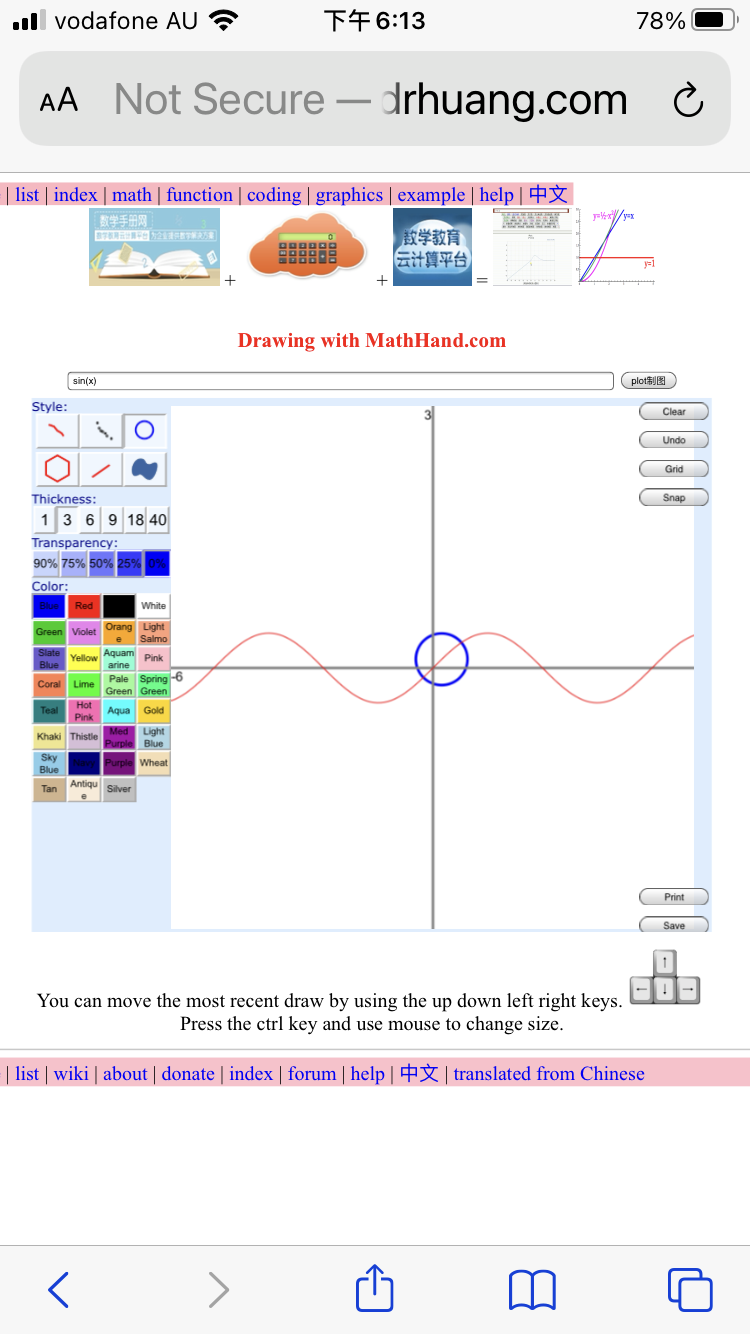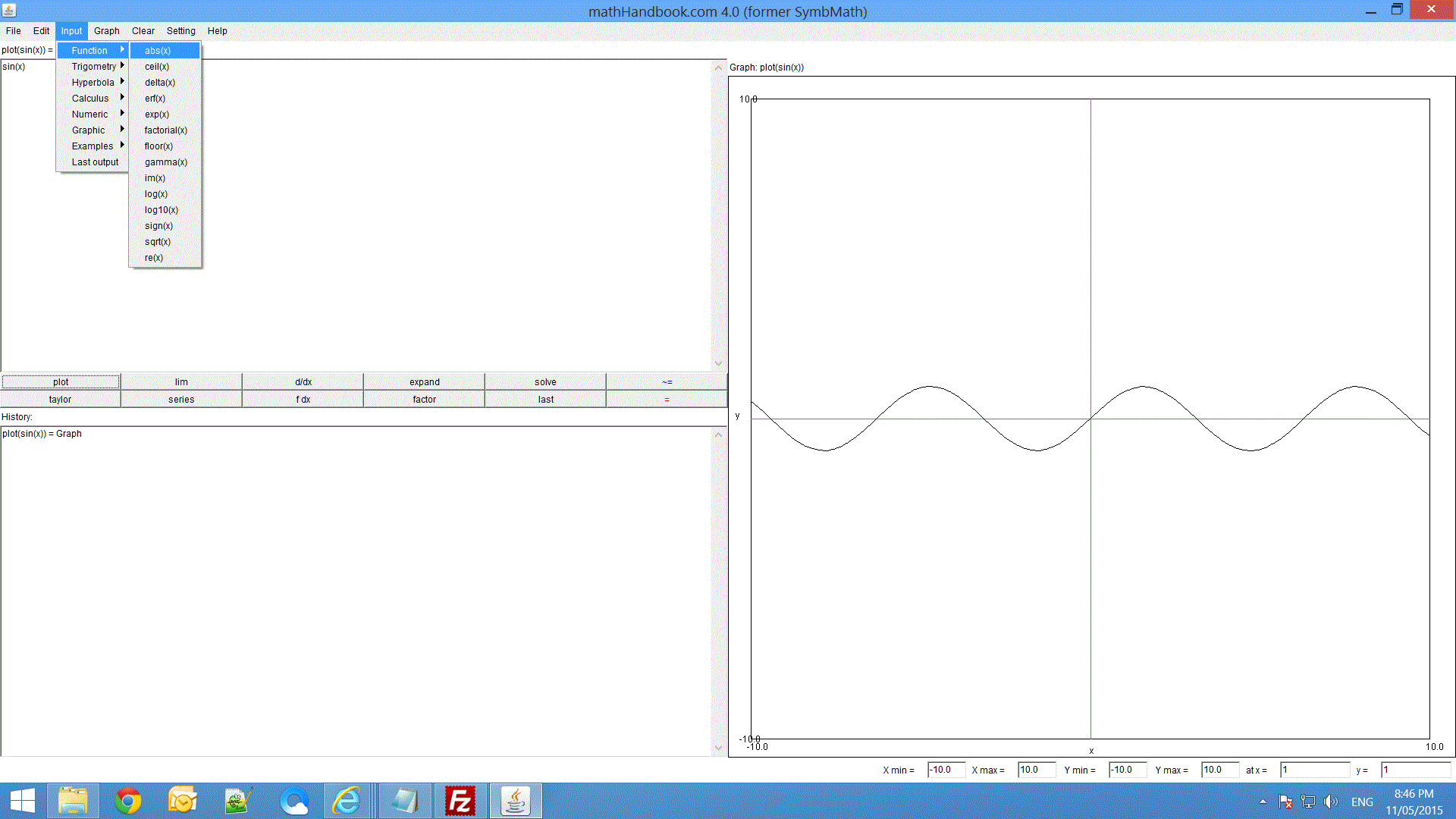﻿ ﻿﻿ math handbook calculator - Fractional Calculus Computer Algebra System software+++=# Math Handbook Calculator

## - Computer Algebra System of Fractional Calculus

programing

zoom graph by mouse wheel

How to use? There are many ways:

1. Input or click sin(x) , click for integration, click button for derivative to check its result, click again for second derivative, click to inverse function, click for definition, click to simplify, click ......
2. Input the unkown y as seond argument,
sin(x)=cos(y), y
then click the button to solve for unknown y.
3. Input command by use of the first function as command, e.g.
then hit the button or the ENTER key in your keybord.
4. Input function, e.g.
5. Input function by use of "," or ";" as separator for multistatements, e.g.
6. Input question mark ? to show index, i.e.
7. Input function and question mark ? to show its function source. e.g.
8. Input function and then click the question mark ? button to show its function graph. e.g.
 To do Button Clear input symbolic answer numeric answer algebra 1st row simplify sin^((0.5))(x) expand (x-1)^2 factor (x^2-1) convert sin(x) to exp(x) convert asin(x) to log(x) convert exp(x) to sin(x) convert sin(x) to sinh(x) convert 2-i to complex() comvert 2-i to complex(2,-1) inverse( sin(x) ) calculus: default variable is x 2nd row convert sin(x) to integral definition differentiate d/dx sin(x) integrate ∫ sin(x) dx infinite integration int_0^oo ( exp(-x) ) dx nth derivative formula d^n/dx^n sin(x) semiderivative d^(0.5)/dx^(0.5) sin(x) semiintegrate d^(-0.5)/dx^(-0.5) sin(x) ODE solve ordinary differential equation for y, ODE( y'-y-x^x=0), PDE solve partial differential equation for y, PDE( ds(y,t)-ds(y,x)=0), test solution for equation and diff equation discrete math: default index variable is k 3th row convert sin(x) to series definition Taylor series expansion taylor(sin(x)) series( sin(x) ) difference Δk^2 Indefinite sum ∑ 1/k^6 partial sum sum_(k=0)^n k partial sum sum_(k=1)^n k infinite sum sum( x^k/k! as k->oo ) infinite sum sum_(k=1)^oo x^k/k fsolve solve functional equation for f(x) f(x+1)-f(x)=x rsolve solve recurrence equation for y(x) y(x+1)-y(x)=x Numeric math 4th row definition( sinh(x) ) limit lim( sin(x)/x as x->0 ) limoo lim( log(x)/x as x->oo ) numeric limit lim _(x->0) sin(x)/x numeric integrate int _0^1 sin(x) dx numeric sum sum _(x=1)^8 x length length(sin(x)) area area(sin(x)) numeric solve equation nsolve( x^2-1=0 ) solve equation for x, solve( exp(x)+exp(-x)=4 ) numeric answer Interactive Plot: zoom by mouse wheel 5th row plot sin(x) and x^2 implicit plot x^2+y^2=1 and x^2+y^2=4 parametric plot parametricplot( x=sin(t) and y=cos(2*t) ) tangent plot tangentplot(sin(x)) secant plot secantplot(sin(x)) integral (sin(x)) polar plot polarplot(sin(4*x)) overlap plot sin(x) Interactive Plot 2D: zoom by mouse wheel 6th row plot2D (sin(x)) show diff and integral curves taylor2D (sin(x)) diff2D (sin(x)) integrate2D (sin(x)) seimd2D (sin(x)) complex2D(sqrt(x)) show real and imaginary curves in real domain ode plot graphically solve y'=sin(x) Interactive Plot 3D: zoom by mouse wheel 7th row plot3D(sin(x)) implicit3D(x*y*z-t) section3D(x*y) contour3D(x*y) spin3D(sin(x)) complex3D(sqrt(x)) show complex function in complex domain Interactive Plot 3D with parameters 8th row surface3D(x*y) plot3DIE in IE plot3DIE(sin(x)) parametric3D(t,t,t) wireframe3D(x*y) Show function graph. Input cos(x) , click the ? button

First line of buttons are for algebra to return function, second line of button are for calculus to return function, third line of buttions are for discrete math with default index variable k to return value, forth line of buttions are for numeric compution to return number. 5th and 6th line of buttions are to plot2D graph. 7th and 8th lines of buttion are to plot3D graph.

The same color buttons are a pair of inverse operators, its result can be checked each other if it returns origial function or not.

## Rules:

put function arguments in parentheses: sin(x) -- correct, sin x -- incorrect; do not omit multiplication: x*sin(x) -- correct, x sin(x) -- incorrect. x**y or pow(x,y) for power. Usual keywords are lowercase, which are different from uppercase, e.g. sin is different from Sin. Its default variable is small letter x, but its default index variable in discrete math is k.

### Example:

• Add new function f(x) = x^2; call f(2)
f(x) = x^2; f(2)

• Add new rule of derivative d/dx f(x_) := sin(x)
d(f(x_), x_) := sin(x);

• Add new rule of integral int f(x) dx := F(x)
integrate(f(x_), x_) := cos(x);

• algebra: convert.
• calculus: limit, nth derivative, differentiation, integral, fractional calculus, convert to integral.
• equation: Inequalities, congruence equation, Dorphine equation, modulus equation, recurrence equation, (fractional) differential equation, (fractional) integral equation, by solve(), dsolve(), lasolve(), rsolve().
• discrete math: sum, partial sum, indefinite sum, infinite sum, convert to sum.
• interactive plot zoom by mouse wheel: polar plot, parametric plot, implicit plot, tangent plot, secant plot, plot, plot2D, plot3D, parametric3D, parametric3Dxy, implicit3D, complex3D, spin3D
• animation:

Please read its example and manual of symbolic computation Computer Algebra System.

## MathHandbook

What is mathHandbook?

the Math Handbook Calculator has the function of machine learning. It is unique in the world to solve the function of any order (such as 0.5 order) differential equations. Enter mathematical formulas on the Mathematics Handbook website, click continuously to calculate calculus, solve equations, give analytical solutions and numerical solutions and diagrams, interactively zoom in the drawing, and zoom in with the mouse wheel. You can use it on your mobile phone to learn computing and development anytime, anywhere.

http://mathhand.com

It has three versions:

1. Phone version: run on any phone online. It does not requires to download anything.3. PC version: DOS version run on PC. Its old name is SymbMath, you can download it.
MathHandbook - Computer Algebra System symbolic computation.

SymbMath - PC DOS version of symbolic computation Computer Algebra System.

### Question?

• bugs post to QQ group 614057790 or forum.
﻿

• ﻿

Ai
airyAi
airyBi
abs
acos
acosh
acot
acoth
acsc
acsch
angle
area
arg
argument
asec
asech
asin
asinh
assume
atan
atan2
atanh
arccos
arccosh
arccot
arccoth
arccsc
arccsch
arcsec
arcsech
arcsin
arcsinh
arctan
arctanh